# Math in Focus Grade 1 Cumulative Review Chapters 7 to 9 Answer Key

Practice the problems of Math in Focus Grade 1 Workbook Answer Key Cumulative Review Chapters 7 to 9 to score better marks in the exam.

## Math in Focus Grade 1 Cumulative Review Chapters 7 to 9 Answer Key

Concepts and Skills

Circle the ten.
Then fill in the blanks.

Question 1.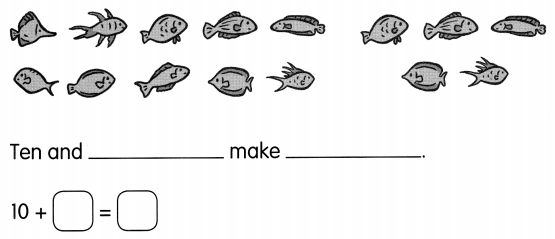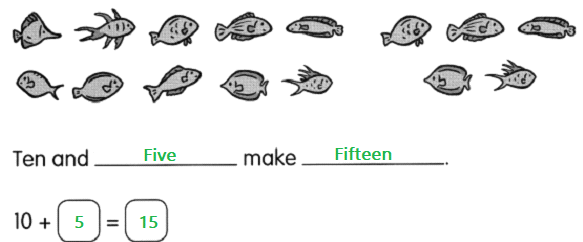Question 2.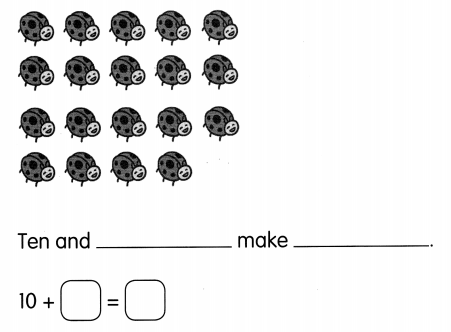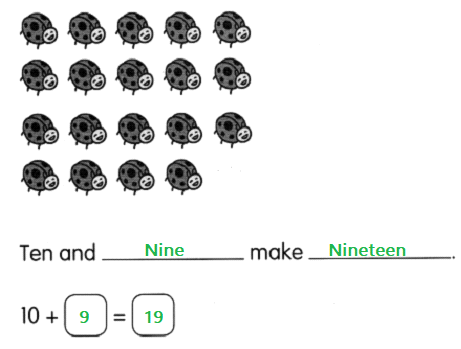Show the number.
Draw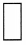for tens and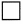for ones.

Question 3.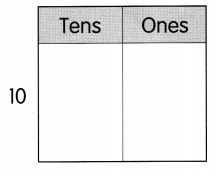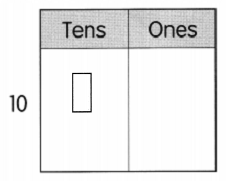Question 4.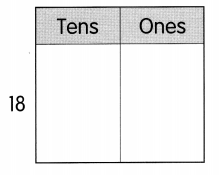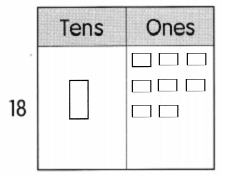Write the number.
Then fill in the blanks.

Question 5.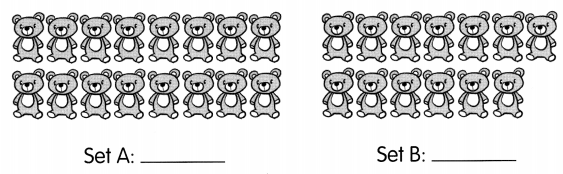Set ____ has ____ more teddy bears than
Set _____
Set A has 3 more teddy bears than
Set B

Compare.
Fill in the blanks.

Question 6.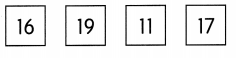____ is the least number.
_____ is the greatest number.
11 is the least number.
19 is the greatest number.

Complete each number pattern.

Question 7.
9, 10, ____, 12, 13, ____, 15
Answer: 9, 10, 11 , 12, 13, 14, 15
Add 1 to each number to get the number pattern.

Question 8.
20, ____, 18, 17, ___, ____, 14, 13
20, 19 , 18, 17, 16 , 15, 14, 13
Add 1 to each number to get the number pattern.

Order the numbers from least to greatest.

Question 9.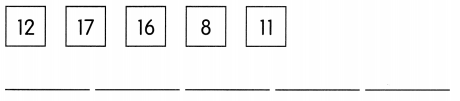Answer: 8, 11, 12, 16, 17

Question 10.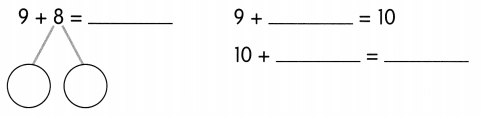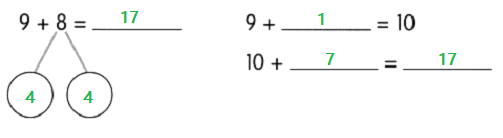Group into a 10 and ones. Then solve.

Question 11.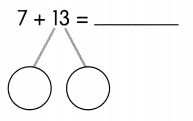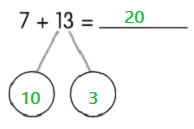Question 12.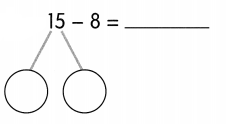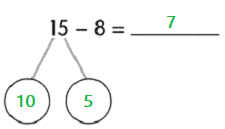Complete.

Question 13.
7 + 7 = ____

Question 14.
7 + 8 = ____

Question 15.
16 – 8 = ____

Question 16.
12 – ___ = 6

Fill in the blanks. Use the words in the box.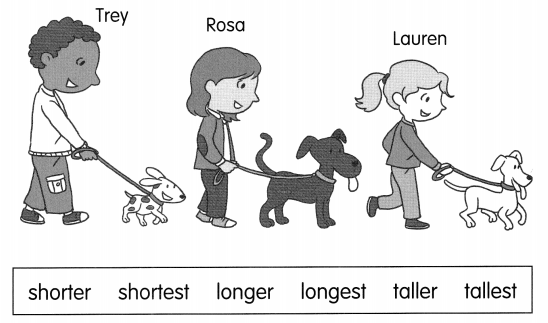Question 17.
Rosa is ____ than Lauren.
Answer: Rosa is taller than Lauren.

Question 18.
Lauren is ___ than Rosa.
Answer: Lauren is shorter than Rosa.

Question 19.
Trey is ___ than Rosa and Lauren. So, Trey is the ____.
Answer: Trey is taller than Rosa and Lauren. So, Trey is the tallest.

Question 20.
The tail on the white dog is ____ than the tail on the spotted dog.
Answer: The tail on the white dog is longer than the tail on the spotted dog.

Question 21.
The tail on the black dog is ____ than the tail on the white dog.
Answer: The tail on the black dog is shorter than the tail on the white dog.

Question 22.
The tail on the spotted dog is the _____.
Answer: The tail on the spotted dog is the shortest.

Complete.

Question 23.
Draw a start line. Then draw a strip that is longer than A and shorter than B.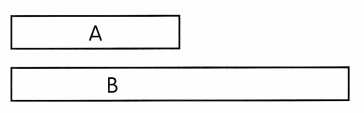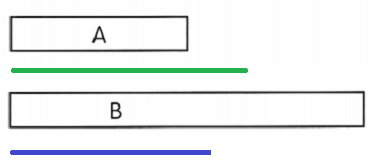Fill in the blanks.

Question 24.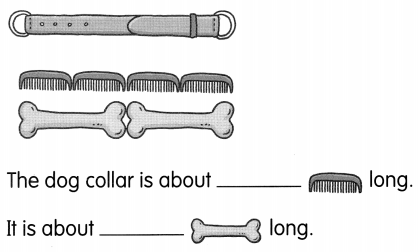The dog collar is about 4 comb long
It is about 2 bone long

Question 25.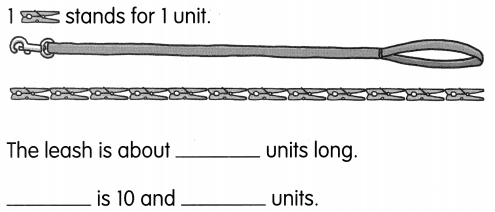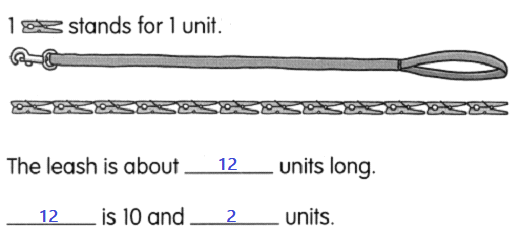Problem Solving

Solve.

Question 26.
Grandma bakes 20 muffins. She gives 8 muffins to Emily. How many muffins does Grandma have left?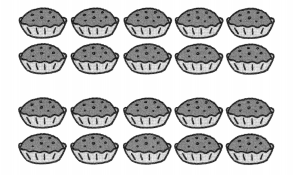_________
Grandma has ____ muffins left.
Given,
Grandma baked 20 muffins,
She gives 8 muffins to Emily,
By subtracting 8 from 20 we get 12,
Therefore, there are 12 muffins left with Grandma.

Question 27.
17 insects are in the garden. 9 are bees. The rest are ladybugs. How many are ladybugs?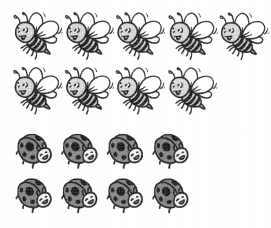_________